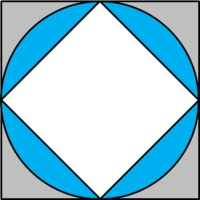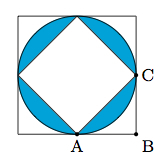# Circle Sandwich

Alignments to Content Standards: 8.G.B.7 8.G.A.2

A square is inscribed in a circle which is inscribed in a square as shown below. Note that the vertices of the inner square meet the midpoints of the outer square's sides.Consider the area of the region left by removing the interior of the small square from the interior of the big square. Is the area of the blue region more or less than $\frac12$ of that?

## IM Commentary

The purpose of this task is to apply knowledge about triangles, circles, and squares in order to calculate and compare two different areas. This can be done in several ways: the solutions below use definition of congruence, the Pythagorean Theorem, and symmetry considerations. The symmetry argument requires auxiliary lines (the two diagonals of the inner square) while the Pythagorean Theorem can be applied to the picture as is after labeling some key points.

This task was adapted from problem #25 on the 2011 American Mathematics Competition (AMC) 8 Test. The question on the exam was different, asking which fraction, from a multiple choice list, is closest to the ratio of the shaded area to the difference of the areas of the two squares: in particular, the exam question could be answered correctly by studying the picture carefully without calculating any of the areas. For the 2011 AMC 8, which was taken by 153,485 students, the multiple choice answers for the problem had the following distribution:

 Choice Answer Percentage of Answers (A)* ${\frac{1}{2}}$ 31.14 (B) ${1}$ 13.94 (C) ${\frac{3}{2}}$ 19.95 (D) ${2}$ 10.14 (E) ${\frac{5}{2}}$ 10.94 Omit - 13.80

Of the 153,485 students: 72,648 or 47% were in 8th grade, 50,433 or 33% were in 7th grade, and the remainder were less than 7th grade.

## Solutions

Solution: 1 Adding and Subtracting Areas

Below is a picture with the two diagonals of the smaller square drawn and some points labelled:The diagonals divide the larger square into 4 smaller, congruent squares because they join the midpoints of the sides of the larger square and reflecting across the vertical one (or horizontal, respectively) exchanges the left side of the big square and the right side (or the top and the bottom, respectively). The sides of the small squares are radii of the circle, so the side lengths are 1 and the area of the small squares is $1^2=1$.

Segment $AC$ is a diagonal of square $ABCD$, and reflecting across the diagonal exchanges triangle $ABC$ and triangle $ADC$ and so these two triangles are congruent. That means that each of the triangles has area $\frac12$. So the small square (which is composed of 4 of these triangles) has area 2, and the area of the big square with the small square removed (shown in grey below) is also 2.The area of the circle of radius $r$ is $\pi r^2$, and since $r=1$, that is just $\pi$. So the blue shaded area is $$\pi - 2 \approx 1.14$$ If we divide the area of the blue shaded region by the area of the grey shaded region in the picture above, we get $$\frac{\pi - 2}{2} \approx \frac{1.14}{2} \gt \frac12$$ In other words, the area of the blue shaded region is a little bit more than half of the area of the grey shaded region.

Since all squares are similar to all squares and all circles are similar to all circles, we can make the same argument with any radius $r$ because the areas of the figure with radius 1 will all scale by a factor of $r^2$. In other words, the area of the circle will be $\pi r^2$, the area of the big square will be $4r^2$, the area of each of the 8 triangles will be $\frac12 r^2$, and the area of the smaller square will be $2r^2$. The area of the blue region will be $\pi r^2 - 2r^2=r^2(\pi-2)$ and if we divide the area of the blue region by the area of the grey region, we get $$\frac{r^2(\pi-2)}{2r^2}=\frac{\pi-2}{2} \approx \frac{1.14}{2} \gt \frac12$$ So the result is the same regardless of the radius of the circle.

Solution: 2 Using the Pythagorean Theorem

Here we apply the Pythagorean Theorem to find the difference of the areas of the two squares. We first focus on finding the side length of the inner square.We know $\triangle ABC$ is a right triangle because we are given that the outer quadrilateral is a square. Let $r$ denote the radius of the circle. We have $|AB| = |CB| = r$ since the side length of the large square is twice the radius of the circle and the small square touches the large square at the midpoints of its sides. By the Pythagorean theorem $$|AB|^2 + |CB|^2 = |AC|^2$$ and so $|AC| = \sqrt{2}r$. This means that the small square has area $\sqrt{2}r \times \sqrt{2}r = 2r^2$. The large square has area $2r \times 2r = 4r^2$. So the difference in the areas of the two squares is $2r^2$.

Instead of using the Pythagorean theorem to find $|AC|$, we could also find the difference of areas of the two squares directly: the inner square together with 4 triangles congruent to $\triangle ABC$ make the outer square and the area of $\triangle ABC$ is $\frac{r^2}{2}$. So the difference of areas of the squares is $2r^2$.

The area of the circle of radius $r$ is $\pi r^2$. So the blue shaded area is $$\pi r^2 - 2r^2 = (\pi -2)r^2.$$ We know that $\pi$ is a little bigger than $3$ so $\pi - 2 \gt 1$ and $$(\pi - 2)r^2 \gt \frac{1}{2} \times 2r^2.$$ This means that the blue shaded area is a little bigger than half of the difference of the areas of the two squares.

Solution: 3 Using Symmetry

Below is a picture with the two diagonals of the smaller square drawn and some points labelled:Note that $\angle ADC$ is a right angle because the diagonals of the small square are perpendicular. So the area of $\triangle ADC$ is $$\frac{1}{2} \times |DA| \times |DC|.$$ Both $|DA|$ and $|DC|$ are radii of the circle. Letting $r$ denote the radius of the circle this means that the area of $\triangle ADC$ is $\frac{r^2}{2}$. If we rotate $\triangle ADC$ about $D$ by 90 degrees, 180 degrees, and 270 degrees, $\triangle ADC$ and its images make up the original small square. So the area of the small square is 4 $\times$ Area($\triangle$ADC) or 2$r^2$.

The area of the circle of radius $r$ is $\pi r^2$. So the blue shaded area is $$\pi r^2 - 2r^2 = (\pi -2)r^2.$$ The area of the big square is $(2r)^2 = 4r^2$ since its sides have the same length as the diameter of the inscribed circle. Removing the inner square from the outer square leaves $4r^2 - 2r^2 = 2r^2$. We know that $\pi$ is a little bigger than $3$ so $\pi - 2 \gt 1$ and $$(\pi - 2)r^2 \gt \frac{1}{2} \times 2r^2.$$ The blue shaded area is a little bigger than half of the difference of the areas of the two squares.